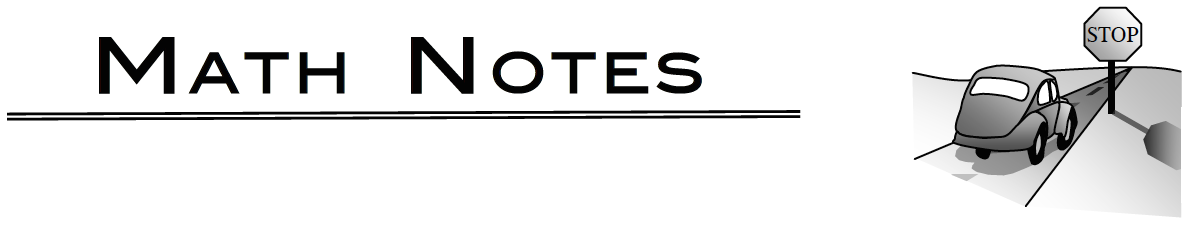### Home > PC3 > Chapter 11 > Lesson 11.1.4 > Problem11-80

11-80.

A golf ball is hit at an angle of $30º$ with an initial velocity of $100$ ft/s. If the ball was struck from the ground, how long was it in the air and how far will it travel down the fairway?## Projectile Motion

An object that is launched with an initial position of $(x_0, y_0)$ and an initial velocity of $v_{0}$ and an angle of $θ$ will travel along a path defined by the parametric function:

$x(t) = (v_0 \cos(θ))t + x_0$

$y(t) = −16t^2 + (v_0 \sin(θ))t + y_0$

where $x$ and $y$ are measured in feet and $t$ is measured in seconds.

First determine how long the ball was in the air, that is, when does the ball hit the ground or have a height of $0$?
This is done by solving $y(t) = 0$.
Once this time is known, it can be used to calculate the horizontal distance traveled.